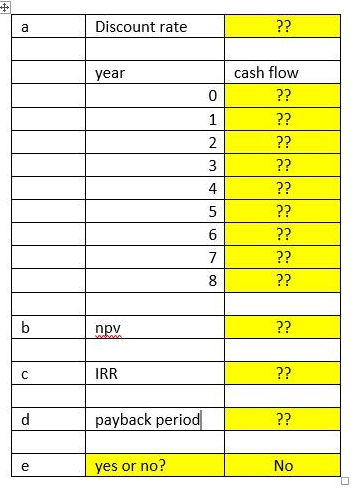# Capital budgeting An investment requires an outlayjagguarpaw January 7, 2017 0 Comments

## Question: Capital budgeting An investment requires an outlay.

Capital budgeting

An investment requires an outlay of \$100,000 today. Cash inflow from the investment are expected to be \$40,000 per year at the end of year 4, 5, 6, 7, and 8. You require a 20% rate of return on this type of investment. Answer the following questions:

First draw the time line and specify the cash outflow and inflow for each period.

Calculate the net present value.

Calculate the Internal rate of return of this investment.

Calculate the payback periods

(please fill out all yellow shaded cells in the table below)